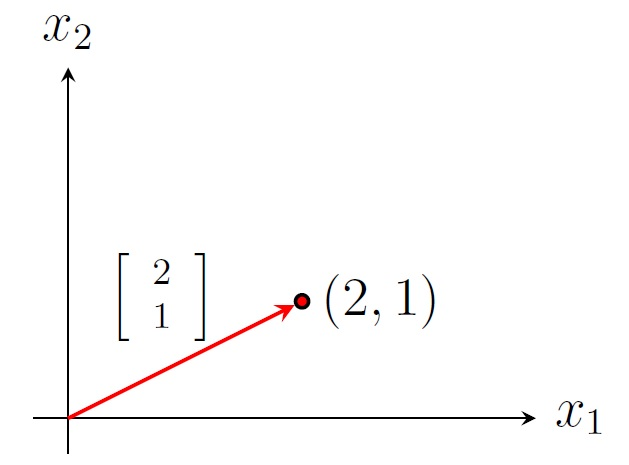Linear Algebra Home

## Matrix Operations

Matrix: An $$m\times n$$ matrix $$A$$ is an $$m$$-by-$$n$$ array of scalars from a field (for example real numbers) of the form $A=\left[\begin{array}{cccc} a_{11}&a_{12}&\cdots &a_{1n}\\ a_{21}&a_{22}&\cdots &a_{2n}\\ \vdots&\vdots&\ddots &\vdots\\ a_{m1}&a_{m2}&\cdots &a_{mn} \end{array}\right].$ The order (or size) of $$A$$ is $$m\times n$$ (read as m by n) if $$A$$ has $$m$$ rows and $$n$$ columns. The $$(i,j)$$-entry of $$A=[a_{i,j}]$$ is $$a_{i,j}$$.

For example, $$A=\left[\begin{array}{rrr}1&2&0\\-3&0&-1\end{array} \right]$$ is a $$2\times 3$$ real matrix. The $$(2,3)$$-entry of $$A$$ is $$-1$$.

Equality: Two matrices $$A$$ and $$B$$ are equal, i.e., $$A=B$$ if $$A$$ and $$B$$ have the same order and the entries of $$A$$ and $$B$$ are the same.

Useful Matrices:

• A zero matrix, denoted by $$O$$ or $$O_{m,n}$$, is an $$m\times n$$ matrix whose all entries are zero.

• A square matrix is a matrix is a matrix whose number of rows and number of columns are the same.

• A diagonal matrix is a square $$n\times n$$ matrix whose nondiagonal entries are zero.

• The identity matrix of order $$n$$, denoted by $$I_n$$, is the $$n\times n$$ diagonal matrix whose diagonal entries are 1. For example, $$I_3=\left[\begin{array}{rrr}1&0&0\\0&1&0\\0&0&1 \end{array} \right]$$ is the $$3\times 3$$ identity matrix.

• An $$n\times 1$$ matrix is called a column matrix or an $$n$$-dimensional (column) vector, denoted by lowercase letters such $$x$$, $$\boldsymbol x$$, or $$\overrightarrow{x}$$. For example, $$\overrightarrow{x}=\left[\begin{array}{r}0\\1\\2 \end{array} \right]$$ is a $$3$$-dimensional vector which represents the position vector of the point $$(0,1,2)$$ in the 3-space $$\mathbb R^3$$ (i.e., a directed line segment from the origin $$(0,0,0)$$ to the point $$(0,1,2)$$).Position vector of a point in the 2-space $$\mathbb R^2$$

Matrix Operations:

Transpose: The transpose of an $$m\times n$$ matrix $$A$$, denoted by $$A^T$$, is an $$n\times m$$ matrix whose columns are corresponding rows of $$A$$, i.e., $$(A^T)_{ij}=A_{ji}$$.
Example. If $$A=\left[\begin{array}{rrr}1&2&0\\-3&0&-1\end{array} \right]$$, then $$A^T=\left[\begin{array}{rr}1&-3\\2&0\\0&-1\end{array} \right]$$.

Properties: Let $$A$$ and $$B$$ be two matrices with appropriate orders. Then

1. $$(A^T)^T=A$$

2. $$(A+B)^T=A^T+B^T$$

3. $$(cA)^T=cA^T$$ for any scalar $$c$$

Scalar Multiplication: Let $$A$$ be a matrix and $$c$$ be a scalar. The scalar multiple, denoted by $$cA$$, is the matrix whose entries are $$c$$ times the corresponding entries of $$A$$.
Example. If $$A=\left[\begin{array}{rrr}1&2&0\\-3&0&-1\end{array} \right]$$, then $$-2A=\left[\begin{array}{rrr}-2&-4&0\\6&0&2\end{array} \right]$$.

Properties: Let $$A$$ and $$B$$ be two matrices of the same order and $$c$$ and $$d$$ be scalars. Then

1. $$c(A+B)=cA+cB$$

2. $$(c+d)A=cA+dA$$

3. $$c(dA)=(cd)A$$

Sum: If $$A$$ and $$B$$ are $$m\times n$$ matrices, then the sum $$A+B$$ is the $$m\times n$$ matrix whose entries are the sum of the corresponding entries of $$A$$ and $$B$$, i.e., $$(A+B)_{ij}=A_{ij}+B_{ij}$$.
Example. If $$A=\left[\begin{array}{rrr}1&2&0\\-3&0&-1\end{array} \right]$$ and $$B=\left[\begin{array}{rrr}0&-2&0\\3&0&2\end{array} \right]$$, then $$A+B=\left[\begin{array}{rrr}1&0&0\\0&0&1\end{array} \right]$$.

Properties: Let $$A,B$$, and $$C$$ be three matrices of the same order. Then

1. $$A+B=B+A$$ (commutative)

2. $$(A+B)+C=A+(B+C)$$ (associative)

3. $$A+O=A$$ (additive identity $$O$$)

Multiplication:
Matrix-vector multiplication: If $$A$$ is an $$m\times n$$ matrix and $$\overrightarrow{x}$$ is an $$n$$-dimensional vector, then their product $$A\overrightarrow{x}$$ is an $$n$$-dimensional vector whose $$(i,1)$$-entry is $$a_{i1}x_1+a_{i2}x_2+\cdots+a_{im}x_n$$, the dot product of the row $$i$$ of $$A$$ and $$\overrightarrow{x}$$. Note that $A\overrightarrow{x}=\left[\begin{array}{c} a_{11}x_1+a_{12}x_2+\cdots+a_{1n}x_n\\ a_{21}x_1+a_{22}x_2+\cdots+a_{2n}x_n\\ \vdots\\ a_{m1}x_1+a_{m2}x_2+\cdots+a_{mn}x_n\end{array}\right] = x_1\left[\begin{array}{c} a_{11}\\ a_{21}\\ \vdots\\ a_{m1} \end{array}\right]+ x_2\left[\begin{array}{c} a_{12}\\ a_{22}\\ \vdots\\ a_{m2} \end{array}\right]+\cdots+ x_n\left[\begin{array}{c} a_{1n}\\ a_{2n}\\ \vdots\\ a_{mn} \end{array}\right].$ Example. If $$A=\left[\begin{array}{rrr}1&2&0\\-3&0&-1\end{array} \right]$$ and $$\overrightarrow{x}=\left[\begin{array}{r}1\\-1\\0\end{array} \right]$$, then $$A\overrightarrow{x}=\left[\begin{array}{r}-1\\-3\end{array} \right]$$ which is a linear combination of first and second columns of $$A$$ with weights $$1$$ and $$-1$$ respectively.

Matrix-matrix multiplication: If $$A$$ is an $$m\times n$$ matrix and $$B$$ is an $$n\times p$$ matrix, then their product $$AB$$ is an $$m\times p$$ matrix whose $$(i,j)$$-entry is the dot product the row $$i$$ of $$A$$ and the column $$j$$ of $$B$$. $(AB)_{ij}=a_{i1}b_{1j}+a_{i2}b_{2j}+\cdots+a_{im}b_{mj}$
Example. For $$A=\left[\begin{array}{rrr}1&2&2\\0&0&2\end{array} \right]$$ and $$B=\left[\begin{array}{rr}2&-2\\0&0\\1&1\end{array} \right]$$, we have $$AB=\left[\begin{array}{rr}4&0\\2&2\end{array} \right].$$

Properties: Let $$A,B$$, and $$C$$ be three matrices of appropriate orders. Then

1. $$(AB)^T=B^T A^T$$

2. $$A(BC)=(AB)C$$ (associative)

3. $$A(B+C)=AB+AC$$ (left-distributive)

4. $$(B+C)A=BA+CA$$ (right-distributive)

5. $$k(AB)=(kA)B=A(kB)$$ for any scalar $$k$$

6. $$I_mA=A=AI_n$$ for any $$m\times n$$ matrix $$A$$ (multiplicative identity $$I$$)

Remark.

1. The column $$i$$ of $$AB$$ is $$A($$column $$i$$ of $$B)$$.
Example. For $$A=\left[\begin{array}{rrr}1&2&2\\0&0&2\end{array} \right]$$ and $$B=\left[\begin{array}{rr}2&-2\\0&0\\1&1\end{array} \right]$$, we have $AB=\left[\begin{array}{rr}4&0\\2&2\end{array} \right] =\left[A\left[\begin{array}{r}2\\0\\1\end{array} \right] \;\;A\left[\begin{array}{r}-2\\0\\1\end{array} \right]\;\right].$

2. $$AB\neq BA$$ in general.
Example. $$\left[\begin{array}{rr}1&2\\3&4\end{array} \right] \left[\begin{array}{rr}0&1\\0&0\end{array} \right]=\left[\begin{array}{rr}0&1\\0&3\end{array} \right] \neq \left[\begin{array}{rr}3&4\\0&0\end{array} \right]=\left[\begin{array}{rr}0&1\\0&0\end{array} \right] \left[\begin{array}{rr}1&2\\3&4\end{array} \right]$$.

3. $$AB= AC$$ does not imply $$B=C$$ in general.
Example. $$\left[\begin{array}{rr}-2&1\\-2&1\end{array} \right] \left[\begin{array}{rr}1&1\\0&0\end{array} \right]=\left[\begin{array}{rr}-2&-2\\-2&-2\end{array} \right]= \left[\begin{array}{rr}-2&1\\-2&1\end{array} \right] \left[\begin{array}{rr}0&0\\-2&-2\end{array} \right]$$.

4. $$AB=O$$ does not imply $$A=O$$ or $$B=O$$ in general.
Example. $$\left[\begin{array}{rr}-2&1\\-2&1\end{array} \right] \left[\begin{array}{rr}1&1\\2&2\end{array} \right]=\left[\begin{array}{rr}0&0\\0&0\end{array} \right]$$.

Powers of a matrix: If $$A$$ is an $$n\times n$$ matrix and $$k$$ is a positive integer, then $$k$$-th power of $$A$$, denoted by $$A^k$$, is the product of $$k$$ copies of $$A$$. We use the convention $$A^0=I_n$$.
Example. $$A=\left[\begin{array}{rr}0&1\\0&0\end{array} \right] \implies A^2=AA=\left[\begin{array}{rr}0&0\\0&0\end{array} \right],\; A^{100}=\left[\begin{array}{rr}0&0\\0&0\end{array} \right].$$

Symmetric and Skew-symmetric Matrices:
A square matrix $$A$$ is symmetric if $$A^T=A$$ and $$A$$ is skew-symmetric if $$A^T=-A$$. A square matrix $$A$$ can be written uniquely as a sum of a symmetric and a skew-symmetric matrix: $A=\frac{1}{2}\left(A+A^T \right)+\frac{1}{2} \left(A-A^T \right)$
Example. $\left[\begin{array}{rr}1&4\\2&5\end{array}\right] =\frac{1}{2}\left( \left[\begin{array}{rr}1&4\\2&5\end{array}\right] + \left[\begin{array}{rr}1&2\\4&5\end{array}\right] \right) +\frac{1}{2}\left( \left[\begin{array}{rr}1&4\\2&5\end{array}\right] - \left[\begin{array}{rr}1&2\\4&5\end{array}\right] \right) =\left[\begin{array}{rr}1&3\\3&5\end{array}\right] +\left[\begin{array}{rr}0&1\\-1&0\end{array}\right].$

Last edited